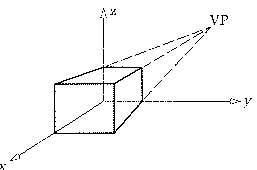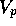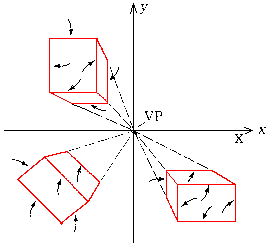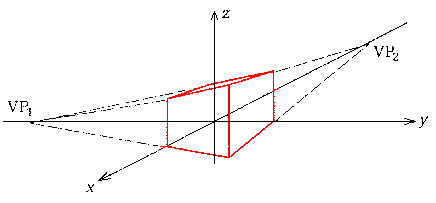Lines not parallel to view plane or receding parallel lines appear to converge at some point called the vanishing point on the view plane. Lines parallel to principal axes (i.e. the world coordinate axes) converge to a principal vanishing point. Different types of perspective projection are named after the finite number of vanishing points. A perspective projection can have 1, 2, or 3 principal vanishing points.

### One point perspective

One point perspective occurs when the projection plane is parallel to two principal axes. Conversely, when the projection plane is perpendicular to one of the principal axis, one point perspective occurs. Receding lines along one of the principal axis converge to a vanishing point.Even though change in eye position or tilt of head (i.e. direction of U and V ) affects the vanishing point, the view is still one point perspective.### Two point perspective

If the projection plane is parallel to one of the principal axes or if the projection plane intersects exactly two principal axes, a two-point perspective projection occurs.### Three point perspective

If the projection plane is not parallel to any principal axis, a three-point projection occursFrom P. Asokarathinam -- see details 27.11.1996